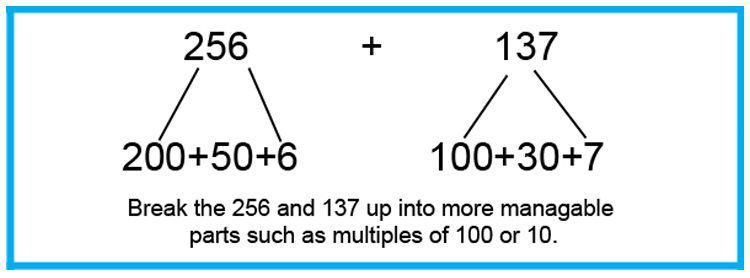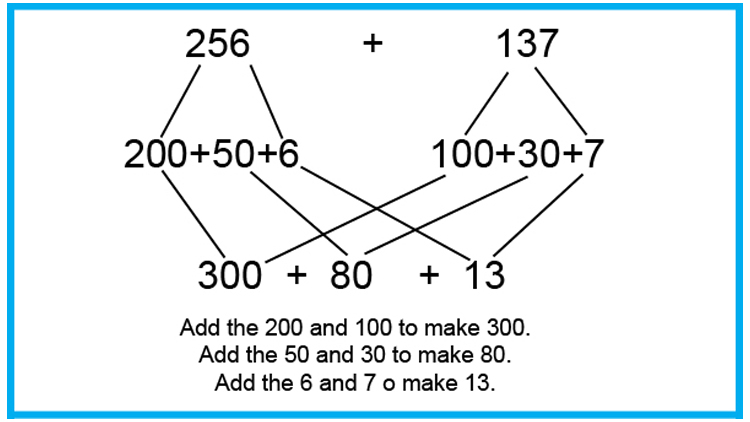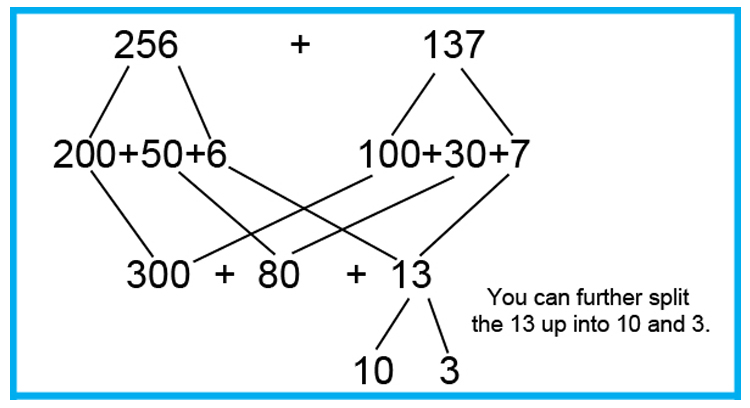# Common core addition - Number bonds

Addition using number bonds uses the concept that “A whole thing is made up of parts”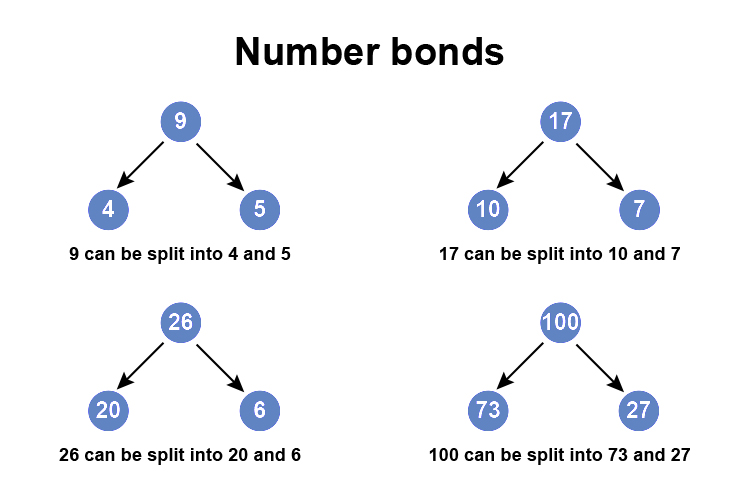Numbers can be broken down in lots of different ways.

Example 1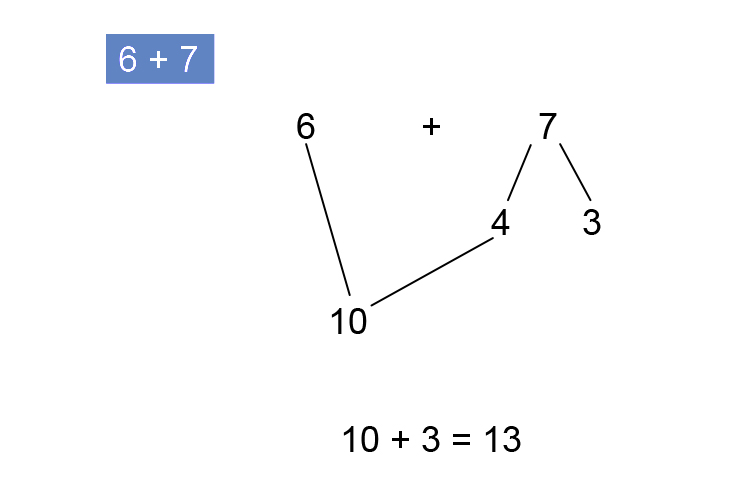NOTE:

Sometimes this is drawn with the circled numbers representing 10 complete.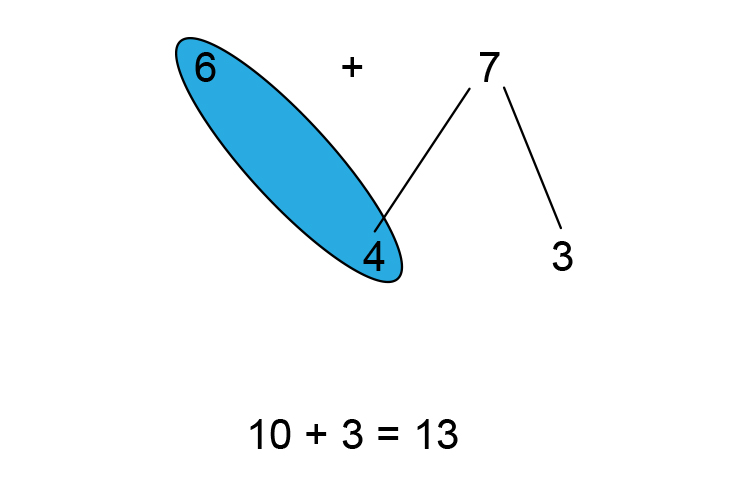To see this in stages

## Step by step

Example 1

6+7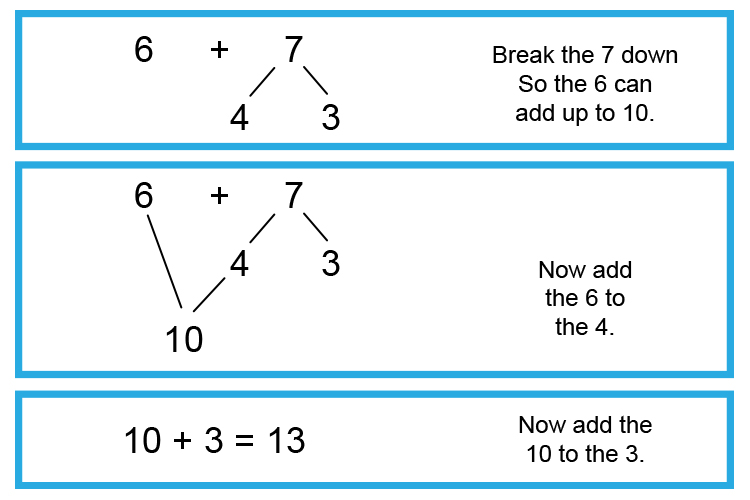Example 2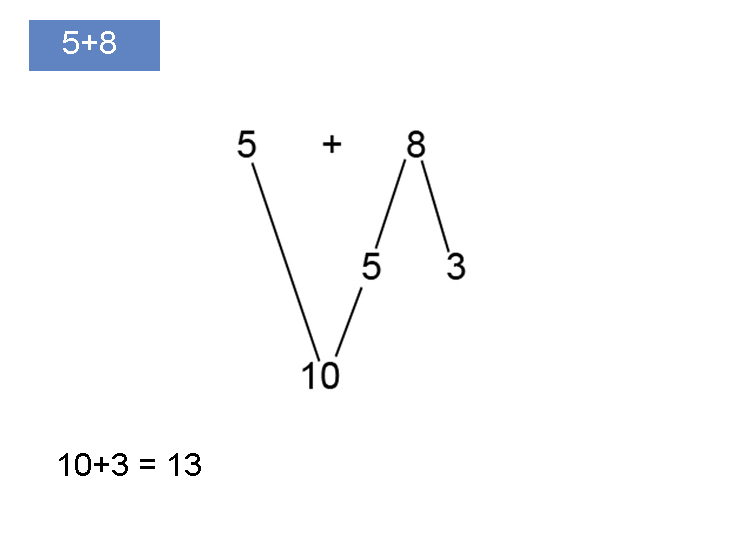To see this in stages

## Step by step

Example 2

5+8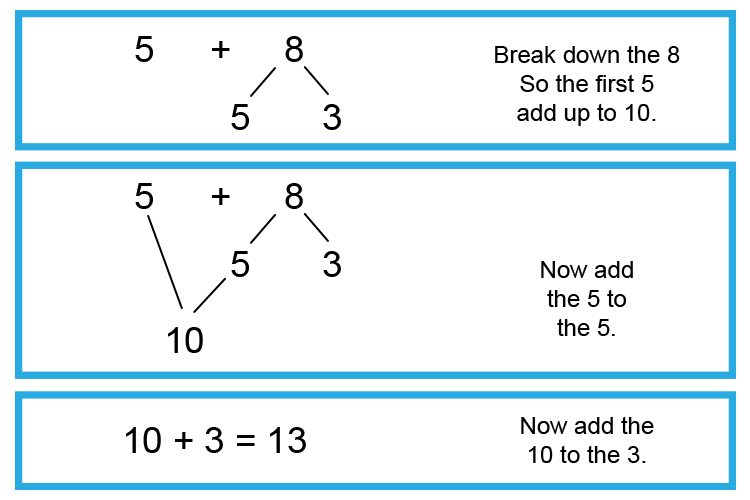Example 3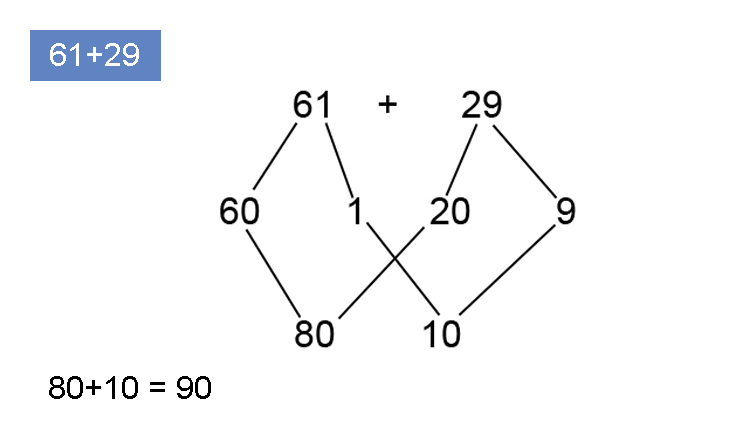To see this in stages

## Step by step

Example 3

61+29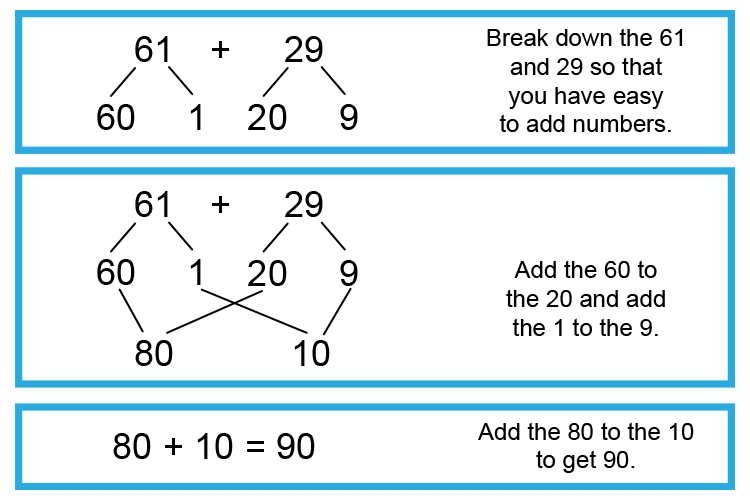Example 4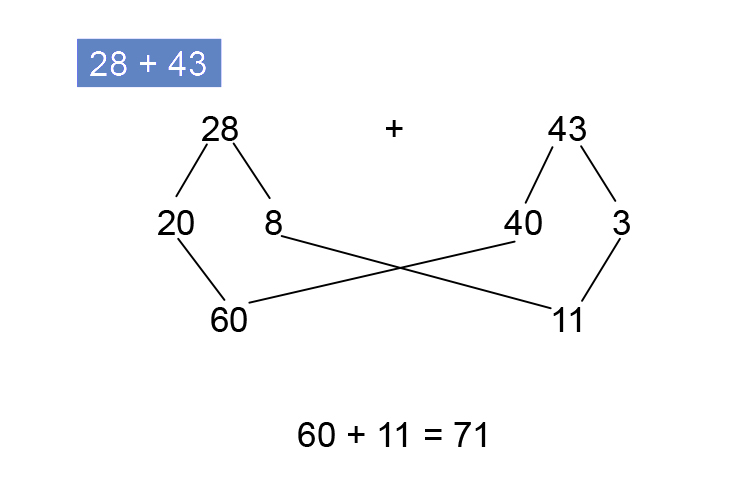To see this in stages

## Step by step

Example 4

28+43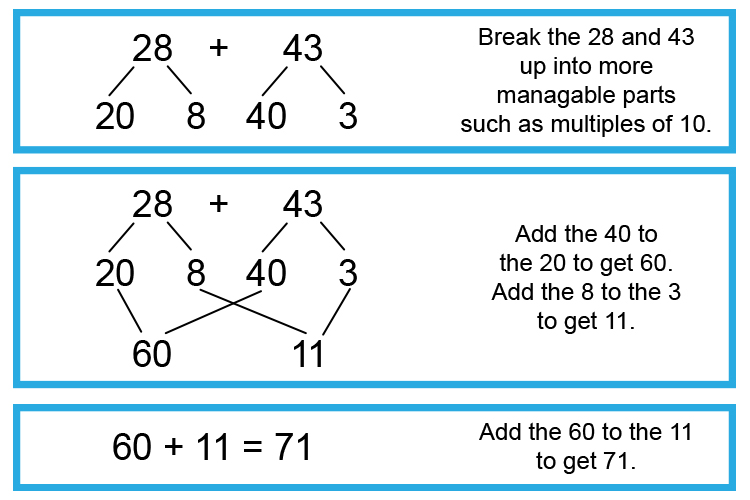Example 5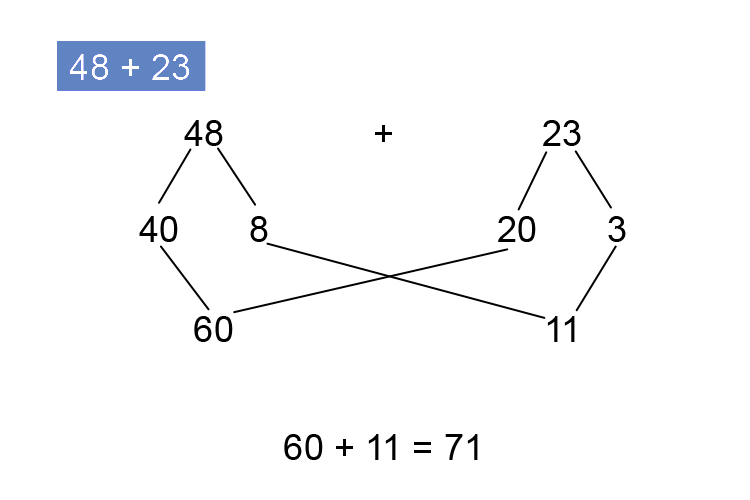To see this in stages

## Step by step

Example 5

48+23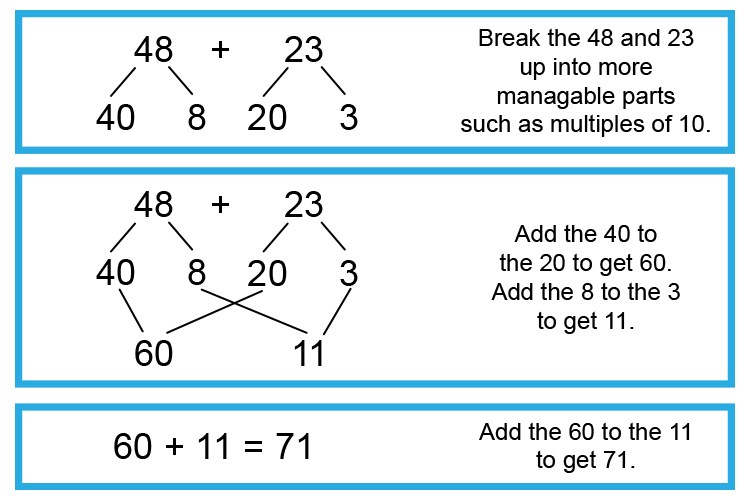Example 6To see this in stages

## Step by step

Example 6

332+85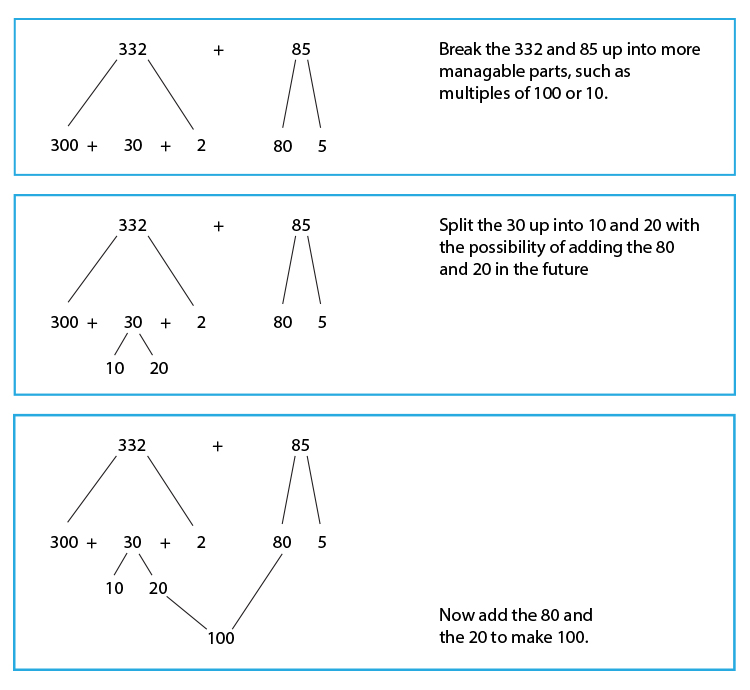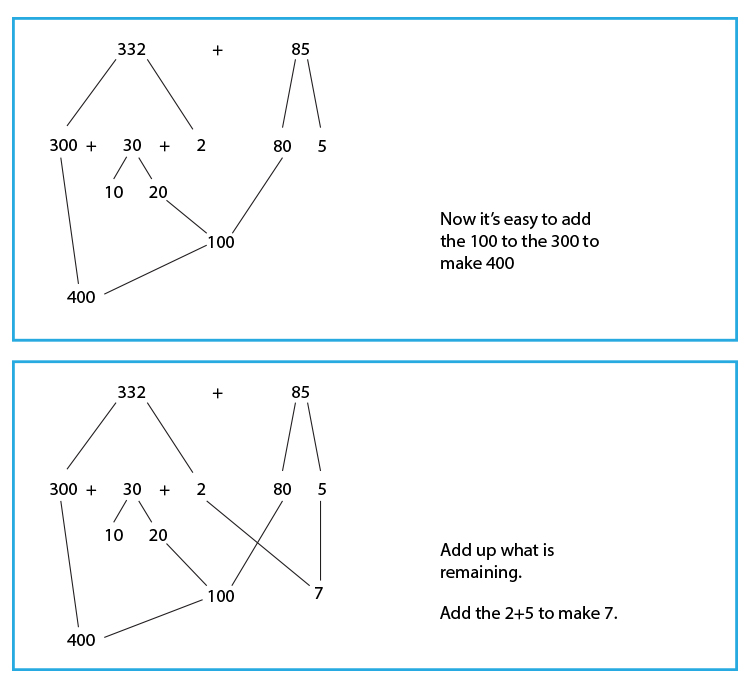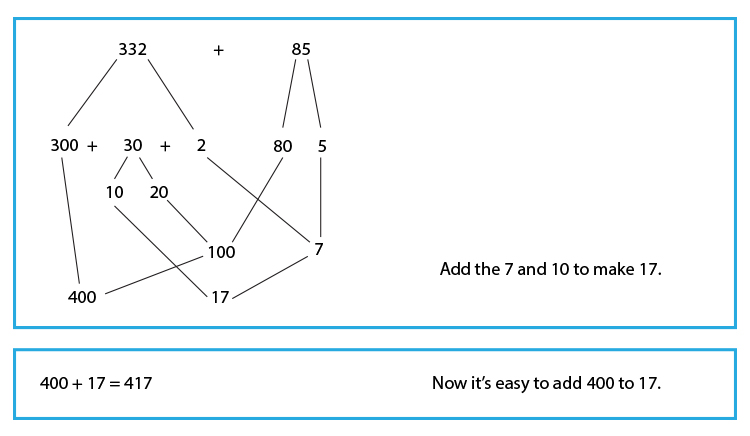Example 7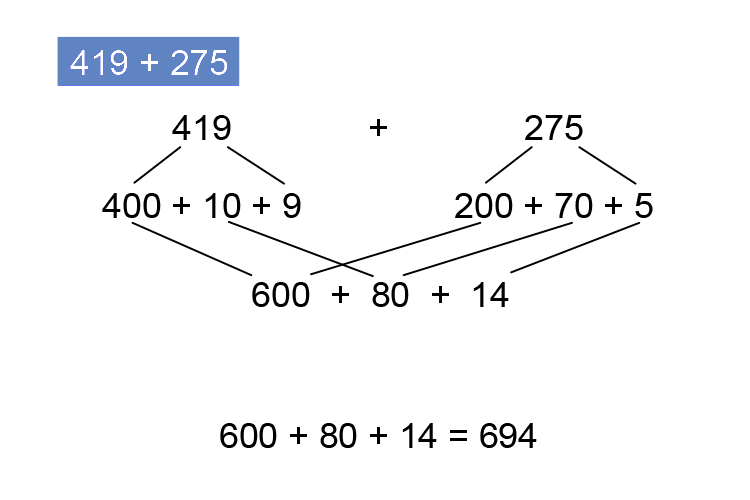To see this in stages

## Step by step

Example 7

419+275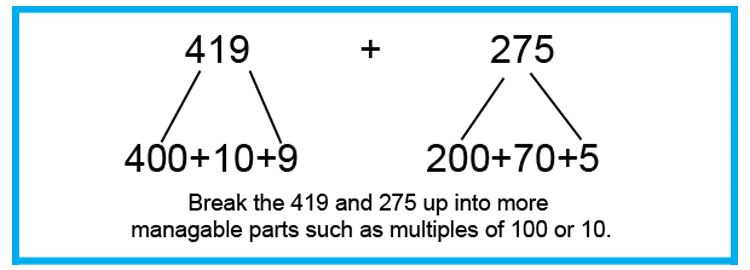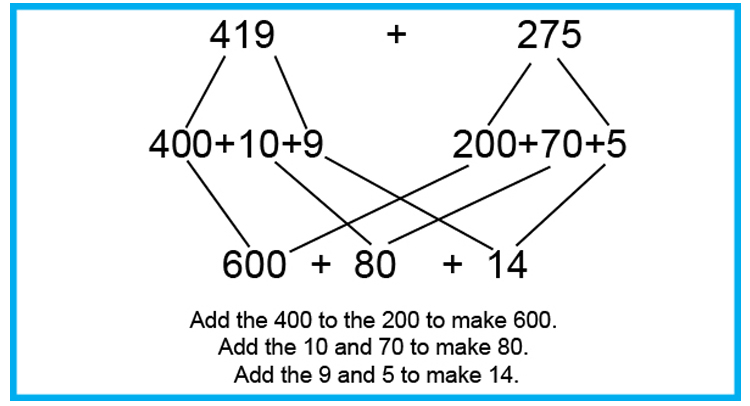Example 8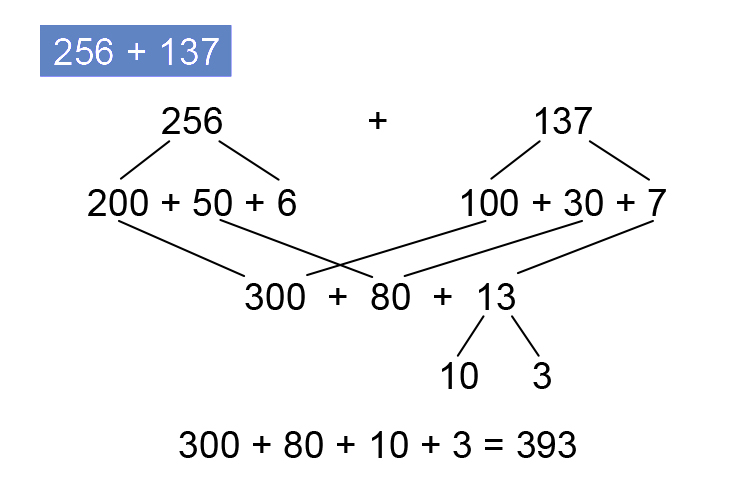To see this in stages

## Step by step

Example 8

256+137